## Introduction

To overcome these bottlenecks, some authors recently suggested the need for a formal mathematical expansion of the additivity formulations that may allow working with differential maximal effects14,15. Other authors have proposed a pragmatic numerical approximation based on a toxic unit extrapolation method to solve the problem5.

Inducible whole cell biosensors are a paradigmatic case of a biological system displaying differential maximal effects and usually biphasic dose-response profiles (e.g.,16) (Fig. 1c). Whole cell biosensors are intact, living cells genetically engineered to produce a dose-dependent measurable signal in response to a specific chemical or physical stimulus in their environment17. Inducible whole cell biosensors response is usually characterized by a dose-dependent biphasic profile presenting an induction region up to a concentration threshold (maximum permissive concentration) and a subsequent inhibition region where the biosensor response decays, possibly due to the inherent toxicity of the analyte above certain concentrations which affects cell viability18. Whole cell biosensors have been extensively used in the last 3 decades for the detection and quantification of different analytes and stresses of interest (for a review see17,19). Despite they have a clear vocation to be applicable in realistic conditions, mixture effect research using inducible whole-cell biosensors is presently a poorly developed research area. From our point of view, the main reason is the lack of a founded theoretical basis and experimental additivity framework.

In the present work, a novel additivity framework for mixture research in the context of whole cell inducible biosensors has been mathematically developed. The method proposes a multivariate extension of the effective dose (EDp) to take into account the occurrence of differential maximal effects and inhibition beyond the MPCs. In effect, this allows an extension of Loewe additivity that enables its direct application in a biphasic dose-response framework. A family of user friendly utilities has been incorporated in the (drc) package20 for R. The method has been illustrated studying the response of the cyanobacterial biosensor Synechoccocus elongatus PCC 7942 pBG2120 to binary mixtures of 6 heavy metals (Zn, Cu, Cd, Ag, Co and Hg). Synechococcus sp. PCC 7942 pBG2120 bears a fusion of the promoter region of the smt locus of Synechococcus sp. PCC 7942 to the luxCDABE operon of Photorhabdus luminescens. It is an inducible self-luminescent whole cell biosensor able to respond to a broad range of heavy metal cations which present differential maximal effects and biphasic dose-response curves16. The method is a useful contribution for the entire whole-cell biosensors discipline and related areas which allows to perform sound mixture-effect research in the framework of biphasic dose-response curves.

## Theory

### A novel framework for mixture-effect research for whole cell biosensors

We propose a novel framework for modelling mixture effects in whole cell biosensors showing biphasic dose-response curves. It is characterized by the following 5 steps: (1) Fitting biphasic dose-response profiles. (2) A dimensional extension of the effective dose notation. (3) Two-dimensional formulation of Loewe additivity. (4) Prediction of mixture effects based on individual component biphasic dose-response data. (5) Analysis of departures from additivity.

### Fitting biphasic dose-response profiles

Inducible whole cell biosensors usually present inverted v-shaped biphasic dose-response profiles (Fig. 1c). This specific type of dose-response pattern may be fitted using nonlinear regression model equations Gaussian and LogGaussian. We considered 2 specific inverted v-shaped functions f: the Gaussian (Eq. 1 below) and the log Gaussian (Eq. 2 below) equations, which are defined as follows: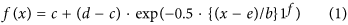where the parameters c and d correspond to the limits for x = 0 and x tending to infinity and the parameters b and e control the steepness of the curve and location of the peak, respectively. The parameter f describes asymmetry in the curve (i.e., asymmetry between the left and right sides of the peak).

### A multivariate extension of the effective dose notation

In the present work, a notation for fractional effective doses EDp scaled from the MPCs (hereafter, Emax) has been chosen (Fig. 2a). The novelty of the approach is that we maintain the empirical effect scale (E(τ)) in the y axis and we project the fractional effect scale (Ep(p)) in the z plane (see Fig. 2a). Fractional effects (p) in the Ep scale are defined as follows: −100 ≤ p ≤ 100. For p < 0, fractional effects on the left side of the Emax are obtained (Fig. 2a) (the induction part of the curve), for p > 0, fractional effects on the right side of the Emax are obtained (the inhibition part of the dose-response curve). p = 0 = Emax = MPC. Decoupling the fractional effect scale (Ep(p)) from the empirical effect (E(τ)) allows to scale inverted v-shaped dose-response curves with differential maximum effects in a unique fractional scale independently of the maximum level of effect (Emax) attained (Fig. 2b). However, EDp needs to be dimensionally extended to account for differences in both the dose (D) and effect (E) scales (Fig. 2b). Therefore we define EDp as a two-dimensional vector (D(p), E(p)) where D(p)is the dose required to get the desired fractional effect (p) (i.e. 50%) and E(p) is the effect in the empirical effect scale (E (τ)) achieved at this fractional effect (p).

### Two-dimensional formulation of Loewe additivity

For classical monotonic dose-response curves, a uni-dimensional effective dose-notation and additivity formulation are enough to perform accurate additive predictions. In the same way that the fractional notation (EDp) needs to be extended to set a proper common fractional effect scale in biphasic dose-response curves, the existing additivity formulation needs to be extended as well. For this we propose a two-dimensional formulation of Loewe additivity computed for the two components of EDp = (D(p), E(p))p (Fig. 2c). The additivity formulation on the dose (D) scale is identical to the original Loewe additivity formulation. For notational convenience, we will formulate hereafter Loewe additivity as follows: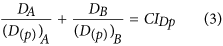where DA and DB are the doses of the components A and B which in combination produces a fractional effect p on the measured biological response. (D(p))A and (D(p))B are the doses that individually result in a fractional effect p for the components A and B, respectively. In case (Eq. 3) equals 1 Loewe additivity holds. Otherwise, Combination Index Theorem holds4. If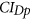denotes the Combination Index in the D dimension (see Fig. 2c) for the considered fractional effect p4,< 1 indicates synergism,> 1 indicates antagonism. To project Loewe additivity to the empirical effect dimension (E(τ)), we simply assume that the effect (E) dimension is equivalent to the Dose (D) dimension in its relationship with the fractional effect (Ep) dimension (Fig. 2c). Therefore, it holds that Loewe additivity is computed in the E dimension as follows: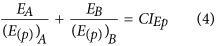where EA and EB are the effects (in the empirical scale (τ)) of the components A and B which in combination results in a fractional effect p on the measured biological response. (E(p))A and (E(p))B are the effects in the empirical effect scale of the components A and B resulting individually in the desired fractional effect p., is the Combination Index in the E dimension for the considered fractional effect p.

The proposed two-dimensional formulations are susceptible of extension to n components as follows: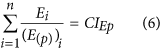where Diis the dose of the ith component which in combination produces a fractional effect p. (D(p))i is the dose of the ith components that individually produces the same fractional effect p. Eiis the effect (τ) of the ith component which in combination produces a fractional effect p. (E(p))i is the effect (τ) of the ith components that individually produce the same fractional effect p. Thus, CIDpand CIEp are the combination index scores for the D and E dimensions at any fractional effect p.

### Predictions of the joint effect of a mixture under two-dimensional Loewe additivity

Equation (5) for CIDp = 1 can be rewritten in a predictive formulation (allowing for in-silico predictions of the joint effect of n mixture components based on individual chemical information only) according to Faust, et al.21, if Di are expressed as relative proportions ji of the total dose (Dmix), where ji = Di/Dmix. Under the condition that the mixture elicits a p total fractional effect, the total dose of the mixture Dmix is defined as the effective dose of the mixture (Dpmix) required to produce a fractional effects p. If CI = 1, Di can be substituted in Eq. (5) by ji Dp mix and by rearrangement, it holds:According to Gonzalez-Pleiter, et al.22, equation (5) can be similarly rewritten as follows when CI ≠ 1: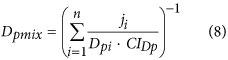Considering the proposed two-dimensional notation EDp = (D(p), D(p)), the formulation of Equation (7) in the E dimension gives: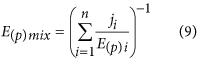In case CI ≠ 1: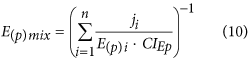### Analysis of departures from additivity

The definition in equations (5) and (6) also allows investigating departures from additivity in biphasic dose-response systems. The result from evaluating equations (5) and (6) are two numerical values of the Combination Index (CI) for the D and E dimensions, which can be expressed as a two-dimensional vector CIp(D, E) = (CID p, CIE p). This approach yields 9 theoretical combinations of CIp (D,E) as follows: CIp (D,E) = (1, 1); (1, <1); (1, >1); (<1, 1); (<1, <1); (<1, >1); (>1, >1); (>1, 1); (>1, <1).

For global assessment of departures from additivity, a simplification of the two-dimensional information can be obtained by calculating a weighted index CIwp for any fractional effect p as follows: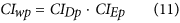where CIwp > 1 indicates overall antagonism, CIwp = 1 an overall additive effect and CIwp <1, an overall synergistic effect.

## Results

Dose-response profiles of Synechoccocus elongatus PCC 7942 pBG2120 exposed to Zn, Cu, Cd, Ag, Co and Hg and their binary combinations were fitted using the proposed set of v-shaped non-linear functions described in Theory section. Best fit models for each metal and metal mixture were selected accordingly to the minimum of the residual sum of squares20. Supplementary Material SM1 shows a summary of the selected model function and parameters for each individual metal and metal mixture. Overall, the majority of single metal experimental data were better fitted by log Gaussian function and only Ag was fitted better by a Gaussian model. On the contrary, the responses of the biosensor to half of the binary metal mixtures were fitted better by Gaussian and the other half by logGaussian model (see Supplementary Material SM1). Figure 3 shows as an example, the goodness of fit of the v-shaped non-linear function logGaussian “lgau2” to Zn response experimental data (see Supplementary Material SM1 for model parameters). As can be observed, the function fits adequately Zn experimental data at both the induction and the inhibition part of the dose-effect curve. Using the drc package we can get EDp vectors (D(p), E(p)) at any desired fractional effect p. For example, we can calculate the Zn ED−50 = (1.50 μM, 40.79 BIF), Zn ED0 = (2.43 μM, 79.31 BIF) and the Zn ED+50 to be (3. 34 μM, 40.79 BIF) (Fig. 3) (definitions in Methods section). Since EDp is two-dimensional, it includes not only the concentration required to produce a specific fractional effect p, but also the actual BIF that the biosensor signal achieves at this specific fractional effect for a specific analyte. A summary of EDp vectors (−50, 0, +50) for the 6 metals can be found in Supplementary Material SM2. As can be seen, the bi-dimensional notation allows to explicitly predict concentrations of the analyte resulting in fractional effects in both the induction and the inhibition regions of the dose-response curves. An illustrative example on how to get EDp vectors can be found in Supplementary Material SM3.

Once individual metal biphasic response profiles are adequately fitted and EDp vectors can be obtained to any fractional effect p, it is possible to predict the biosensor response to any combination of the individual metals evaluating Equations (7) and (9). However, prior to any further analysis, it is required to validate the proposed two-dimensional definition of Loewe additivity. Figure 4 shows predicted vs experimental dose-response patterns of sham binary mixtures for Zn:Zn, Cd:Cd and Cu:Cu. A near perfect overlap occurred between the experimental and predicted dose-response curves of sham mixtures for the 3 metals. This validates the proposed multivariative formulation of Loewe additivity model for biphasic dose-response curves, even at mixture concentrations where inhibition of the signal occurs (See Fig. 1b).

Once the multivariative extension of Loewe additivity was validated with the sham mixtures, we applied the method to perform additivity predictions and to study the nature of the interaction (if any) of the response of Synechoccocus elongatus PCC 7942 pBG2120 to the 15 possible binary mixtures of Zn, Cd, Cu, Ag, Hg and Co. Complete results of the analysis can be found in Supplementary Material SM5. A representative selection of the results is presented in Fig. 5. In the case of the binary mixture Cu:Zn (Fig. 5a), the additivity prediction fitted reasonably well the experimental dose-response profile. However, the additivity predictions of the binary mixtures Zn:Cd (Fig. 5b) and Zn:Co (Fig. 5c), deviated from their respective experimental dose-response pattern, suggesting a departure from additivity. However, which kind of departure are we observing?

To answer this question, a quantitative analysis of the nature of the interactions is required3,4. Departures from additivity can be quantified solving Equations 5 and 6 for any fractional effect p. As illustrative example, we used extended p-CI plots to graphically present the results of the quantification of the interactions of the three selected mixtures (Cu:Zn, Zn:Cd and Zn:Co). Extended p-CI plots allow to observe the nature of the interactions along the D and E dimensions covering the complete biphasic dose-response curves, representing the deviation from the additivity line (CI = 1) for any fractional effect p. Figures 5d,f,h and Figure 5e,g,i show extended p-CI plots for the D and E dimensions (respectively), for the three selected metal binary mixtures (Cu:Zn, Zn:Cd and Zn:Co). Extended p-CI plots for D and E axis (Fig. 5d,e, respectively) for the Cu:Zn combination showed CI values near 1 (the additivity line) along the entire range of fractional effects (p) in both dimensions, confirming the near additive behaviour of this metal combination. The Zn:Cd binary combination presented statistically significant synergism (CI <1, p < 0.05) along the entire range of fractional effects (p) in the D dimension (Fig. 5f), but statistically significant antagonism (CI >1, p < 0.05) in the E dimension (Fig. 5g). On the other hand, the Zn:Co combination showed a p-dependent interaction patters in the dose (D) axis (going from antagonistic to synergistic) and consistent synergism (CI <1, p < 0.05) in the effect (E) axis along the entire range of effect levels (p) (Fig. 5h,i). See Supplementary Material SM4 for specific p-values for three selected fractional effect levels (−50, 0, +50). Figure 5j–l showed Extended p-CIw plots for the mixtures Cu:Zn, Zn:Cd and Zn:Co, respectively. Extended p-CIw plots (see equation 11) can be used as a measure of the overall fitness to additivity of the response of a biosensor to mixtures of analytes. As can be seen in the Fig. 5j, the overall effect of the combination of Cu:Zn combination was additive, that of Zn:Cd combination was p-dependent (Fig. 5k), going from synergistic (below the MPC) to antagonistic (above the MPC). That of Zn:Co was consistently synergistic CI < 0.5 along the entire range of effect levels (p) (Fig. 5l).

The effect of the metal ratio on the predictive power of the multivariative extension of Loewe additivity was addressed for selected binary mixtures. Figure 6a–c shows the experimental dose-response patterns and the respective additivity predictions for the three different metal ratios (75:25, 50:50, 25:75, respectively) of the binary metal mixture Cu:Zn. Extended p-CI plots for the D and E dimensions for the different metal ratios are presented in Fig. 6d–i. In addition, extended p-CIw plots are presented in Fig. 6j–l. The selection of metal ratios allows to specifically address the possibility of predicting the dose response patterns including metal combinations in which eventually one of the metal may be present below the MPC and the other above the MPC and vice versa. As can be seen in Fig. 6, the main features of the dose-response pattern of the 50:50 mixture of Cu:Zn (Fig. 6b), that is additivity in D, synergism in E and overall additive effect (CIw > 0.5) is essentially conserved in the 25:75 and the 75:25 ratio. The only differences were the occurrence of a slight tendency to synergism in D in the 25:75 ratio (Fig. 6h) and a slight tendency to synergism in both the 75:25 and 25:75 ratios based on CIw (but still additive based on the management criterion: 0.5 < CIw < 2). Similar results were obtained for ratio variations for the mixture Zn:Cd (Supplementary Material SM4).

The analysis of the departures from additivity for the 15 possible binary combinations of the studied metals revealed a complex scenario where the 9 possible theoretical combinations of the CID,E vectors anticipated in Theory section were actually found (Supplementary Material SM5). In order to get a global idea on the fitness to additivity of the response of the biosensor to the binary mixtures of metals, we computed CIw values according to Equation (11) which were summarized as well as polygonograms4 (for p levels −50, 0, +50) in Fig. 7. Interestingly, additive or near additive effects (according to the management criterion: 0.5 < CIw < 2) hold for binary mixtures of Zn, Cd, Ag and Cu at the three representative p levels (Fig. 7). However, some mixtures containing Hg, Co and Ag resulted in significant departures from additivity: The mixtures Hg:Co and Hg:Ag resulted in synergism and antagonism, respectively at the three representative p levels (−50, 0, +50). In addition, some mixtures resulted in effect-level dependent departures from additivity: the mixture Co:Zn resulted in synergism at p = 0 and p = +50 and the mixture Ag:Cd resulted in synergism at p = +50.

## Discussion

Here we present a theoretical framework which allows to perform sound mixture-effect research in inducible whole cell biosensors and related fields. To have a mathematical formulation of additivity is crucial in order to obtain accurate and comprehensive results in mixture research as demonstrated in the last 20 years in pharmacology and eco/toxicology2,3,4. In these disciplines, Loewe additivity is the gold standard for additivity formulations2,3,4. However, the practical applicability of Loewe additivity is historically hampered in biological systems presenting differential maximal effects and non-monotonic responses such as biphasic dose-response curves5,14. In the present work we propose a multivaritive extension of Loewe additivity which allows its application in the context of differential maximal effects and biphasic dose-response curves. The proposed methodology was validated and tested using the inducible whole-cell self-luminescent metal biosensor Synecchococus elongatus PCC 7942 pBG2120 as case study. Our solution is in agreement with the conceptual formulations which Belz, et al.14 proposed in order to extend the applicability of Loewe additivity in the context of hormesis (an stimulatory effect found at low doses of toxicants). They postulated that differential maximal hormetic effects among individual mixture components force the need to perform additivity formulations in both dose (D) and effect (E) dimensions independently. As solution, they proposed to use the original Loewe equation (Equ. 3) for predictions in the (D) dimension, but a simple summation of fractional effects for predictions in the E dimension. Their predictions were reasonable for the dose (D) dimension, but were, in their own words “more dubious” for the (E) dimension14. This is because their proposal of Loewe additivity formulation for the E dimension was not accurate. We have demonstrated with the sham mixtures that our definition (Eqs 4 and 6) is a true projection of Loewe additivity in the (E) dimension. Scholze, et al.5 recently proposed an elegant approach to the differential maximal effect problem. Since, without multivariate extension, it is impossible to solve Loewe additivity equation for systems presenting differential maximal effects, they proposed a numerical approximation as a solution. Basically, they constructed an extrapolated interval of mixture predicted effects based on reasonable maximal and minimal hypothetical contributions of the less potent mixture components. The method worked well with a biosensor based on partial agonists of aryl hydrocarbon receptor (AhR) used for mixtures including components with varying maximal effects. However, they recognized that it cannot work in systems presenting inhibitory thresholds beyond the MPCs5.

The availability of a Loewe additivity formulation conceived for inducible whole-cell biosensors is an important milestone in this field of research which may allow for a wider generalization of mixture-effect research. This is especially true if a user friendly utility is available. We have made available all the presented mathematical equations, statistical and graphical utilities in the “dose-response curve” (drc) package for R20. Currently the methodology is presently set up for binary mixtures and only two biphasic models (Gaussian and logGaussian) are available. However, future work will focus on extending the framework to n-component mixtures and more biphasic models.

## Conclusions

A multivariative extension of the effective dose (EDp) notation in order to take into account the occurrence of differential maximal effects and signal inhibition beyond the MPCs in biphasic dose-response curves has been developed. This allows a multivariative extension of Loewe additivity enabling its direct application in a biphasic dose-response framework. The proposed additivity definition has been experimentally validated using sham mixtures, finding excellent agreement between experimental and predicted dose-response patterns. The method was applied to study the response of the cyanobacterial self-luminescent metallothionein-based whole-cell biosensor Synechoccocus elongatus PCC 7942 pBG2120 to binary mixtures of 6 heavy metals (Zn, Cu, Cd, Ag, Co and Hg). The response of Synechoccocus to the mixtures can be considered nearly additive except when Hg, Co and to lesser extent Ag were present in the mixtures which resulted in important departures from additivity. The method has different applications and is a useful contribution for the entire whole-cell biosensors field and related areas allowing to perform sound mixture research in non-monotonic dose-response frameworks.

## Material and Methods

### Chemicals

Chemicals were of analytical grade, culture media and heavy metals stock solutions were prepared in MilliQ water. Heavy metal salts ZnCl2, CdCl2, AgSO4, CuSO4, HgCl2, CoCl2, PbNO3, MgCl2, NiCl2, FeCl2, BaCl2 and SrCl2 were from Sigma-Aldrich (Germany). Concentrated metal salts solutions (1000 mg/L) were prepared in deionized water (Millipore) and stored at 4 °C in opaque bottles. Dilutions and mixtures were freshly prepared in MilliQ water before the experiments.

### Heavy metal mixture exposure experiments

Culture conditions and heavy metal exposure of self-luminescent Synechoccocus elongatus PCC 7942 pBG2120 were as previously described16. Briefly, exposure was performed in transparent 24 well microtiter plates in 1.5 mL final volume BG11 medium (without Co, Ni, Cu, Zn). Plates were incubated at 28 °C in the light, Ca. 40 μmol photons m2 s−1 during 4 h. Luminescence measurements were performed in a Centro LB 960 luminometer in opaque white 96 well microtiter plates. The biosensor signal was expressed as luminescence induction factor (BIF) as previously reported16,28.

Mixture exposure design was adapted from3,29. Basically, Synechoccocus elongatus PCC 7942 pBG2120 was exposed to Zn, Cu, Cd, Ag, Co and Hg and to their 15 possible binary combinations. Binary mixtures were prepared according to a constant ratio design (1:1) based on the individual D−50 (the dose required to get half of the MPC of each individual metal). 7 to 9 serial dilutions (factor 2) of each individual metal and binary combination were tested at the same time4. At least three independent experiments were performed.

### Experimental validation of the additivity definition by sham mixtures

Validation of the additivity definition was performed according to the sham mixture procedure30. sham mixtures are false mixtures in which the two components are exactly the same substance and therefore, their fractional combination should be perfect additivity30. If an additivity formulation is correct, experimental and predicted dose-response pattern of the sham mixtures should perfectly overlap. sham mixtures of Cu:Cu, Zn:Zn and Cd:Cd (ratio 1:1) were prepared and tested as described in theory.

### Analysis of results

#### Fitting biphasic dose-response profiles and obtaining EDp vectors

The entire dose-response profiles of the response of Synechoccocus elongatus PCC 7942 pBG2120 to the different individual heavy metal cations (Zn, Cu, Cd, Ag, Co and Hg) and their binary combinations were fitted using the non-linear functions described in Theory, with and without the use of variance-stabilizing Box-Cox transform-both-sides approach20. Best fit models were selected based on the minimum of the residual sum of squares20. Effective doses (EDp) = (D(p), E(p)) for the different metal cations and combinations were calculated from the fitted v-shaped nonlinear functions.